Document Type : Original Research Article

Author

Department of Engineering, Sari Branch, Islamic Azad University, Sari, Iran

Abstract

Electricity storage is one of the most important issues in the electricity industry, which can generate many ancillary services such as load curve leveling, peak shaving, integration with renewable units, rotating reservations, etc. This paper presents a new and practical method for locating, sizing, and optimally planning vanadium current storage batteries in the above distribution substations. In this innovative method, using load forecasting, the amount of peak consumption per day is calculated, and based on it, several indicators are defined to prioritize the storage installation location. Then, according to the power consumption information in the selected substation, the optimal storage capacity for leveling the load curve is determined, and finally, optimal planning for charging and discharging the storage is presented. In this paper, for simulation of real consumption data of the above distribution network of Semnan city has been used.

Graphical AbstractKeywords

Main Subjects

###### ##### Full Text

1. Introduction

This paper will be very useful for distribution networks that want to do studies related to storage installation and production planning to increase productivity and can be used for all distribution substations. The method presented in this paper has been implemented on the actual consumption data of the distribution substations in Semnan city.

In this paper, the main purpose of the storage curve is to level the load curve. Because the storage location of the above distribution substations is 63.20 kV and the nominal power of these substations is usually several megawatts of amps, so the selector storage should be able to store part of this energy. Have. Among the storage devices, due to the nature of the distribution substations and the space available, the most suitable option is a vanadium flow battery. In flow batteries, the electrolyte is present in two separate tanks, and the reactions take place in a separate cell divided into two parts by a membrane. In these batteries, the electrolyte is pumped from two tanks by a pump to the cell in which the chemical reaction takes place. These batteries work like hydrogen fuel cells, which are made up of two electrolytes stored in two separate tanks. Figure (1) shows the structure of a current battery. Among flow batteries, vanadium batteries are the most widely used. Charging and discharging efficiencies of these batteries are usually between 80 and 85%, their life cycle is usually above 10,000 cycles, energy density between 30 to 50 watts per kilogram, and power density of 80 to 150 watts per kilogram. The most important advantages of these batteries, in addition to high power and energy capacity, are long life due to easy electrolyte replacement, full discharge capability, low spontaneous discharge, and low operating temperature. Hence, the use of vanadium flow batteries is growing rapidly [22-20].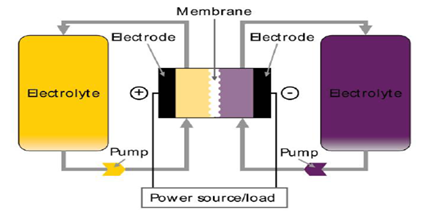Figure 1: Vanadium flow battery structure

3- Modifying the daily consumption curve

The daily consumption curve shows the amount of demand consumed per hour of the day. Consumption continuity curve is an ordered daily consumption curve from the highest consumption hours to the lowest consumption hours and indicates the amount of load continuity based on time. The flatter the consumption curve, the more desirable it is from the point of view of the consumption network. Usually in power grids, load profile correction is an important issue that is done by peak shaving and leveling the load curve. Peak shaving is the elimination of the peak consumption value from the manufacturer's point of view and leveling the load curve is reducing the difference between the maximum and minimum value in the consumption curve. Using the storage device, energy can be stored during off-peak hours and delivered to the grid during peak hours. This reduces the network peak, which can be useful in two ways; first, having a significant peak in the network is not economical, because all network equipment must be upgraded to peak power. Therefore, installing a storage device can delay network development [23-27] and secondly, consumers who are fed during peak hours have to incur higher costs and energy is more expensive. Buy more. Therefore, installing a storage device for peak shaving and leveling the load curve is in the interest of both the network owners and the energy consumers. For example, if the price of energy at i hour is equal to Pri, the amount of profit obtained from storing energy at cheap hours and selling it at expensive hours is obtained by Equation (1) [28-30].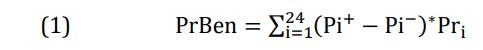In relation (1), if the storage is in charge mode, the value of Pi+ is zero, and if it is in discharge mode, the value of Pi- is equal to zero.

Due to the different electricity tariffs at different hours, Pr Ben will be a significant amount. In the discussion of delaying network development, if the annual load demand grows at the rate of τ% and the storage can reduce the network peak by α%, then the time in years in which the storage providers delay development The network is obtained according to Equation (2) [31-33].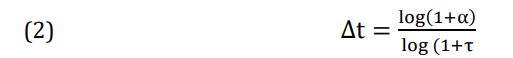Considering the deposit interest and inflation rate, significant profits can be made by postponing the development of the network and postponing the investment for up to eight years. The profit resulting from this delay will be calculated by Equation (3) . In relation (3), Cinv is the cost of network development, ir is the inflation rate and dr is the deposit interest rate.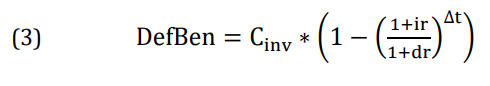4- Select the best post to install the storage

In 20/63 kV substations, due to the independence of the substations from each other, it is possible to have a suitable program for selecting the installation location of the battery and planning its charging and discharging. In terms of picnic characteristics and load curve leveling, the best place to install is the postal storage, which has the most changes during the consumption period or needs to be upgraded shortly; So that the storage can reduce these changes or delay its development. Usually, the peak consumption in Iran is in summer. In recent years, network peaks usually occur in mid-summer, between 2 and 4 pm on non-holiday days [34-36]. Figure (2) shows the power consumption curve of the whole country in the peak week (August 1-7) related to the year 2014.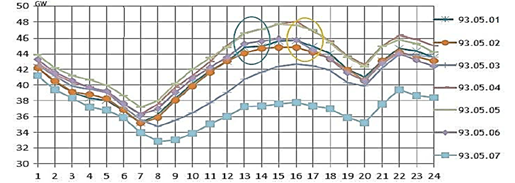Figure 2: The country's electricity consumption curve (peak week) in 2014 

By forecasting the load in the summer and finding the peak consumption, it is possible to make optimal planning for peak loading and leveling. Also, three indicators are considered to determine the installation location of the storage device. The first index is related to the peak, the second index is related to the leveling of the load curve and the third index is related to the reduction of costs and the increase of profits, which are explained below.

4-1- Indicators related to peak shaving

According to the different capacities of the substations and for more simplicity, the consumption values of each substation are divided by the maximum consumption amount in that day so that all consumption continuity curves are per unit. To obtain this index, first the average and standard deviation of the per unit consumption continuity curve are calculated, then according to the consumption continuity curve, the indices are defined as follows [37-39]:

- Kup: The number of hours consumed is more than average.

- Hup: The difference between the maximum consumption (1 per unit) and the average amount.

- Sup: The sum of the difference between the consumption continuity curve and the average up to Kup-th hours

- Stdup: The standard deviation of the difference between the consumption curve and the average up to the Kup-th hours.

Figure (3) shows the parameters defined in the interface.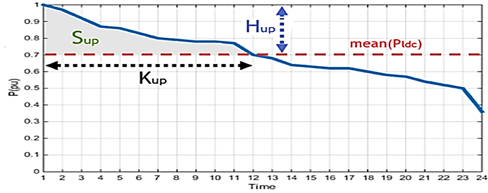Figure 3: Indicators on the consumption continuity curve

According to the above parameters, the first peak shaving index is defined as follows: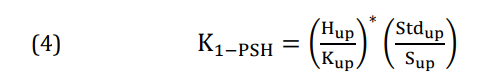In the above relation (Hup/Kup) indicates the average slope of the reduction of the consumption continuity curve, which the higher the value of this slope indicates that the curve is more suitable for peak shaving, because the higher the slope, the more This indicates that peak hours are more different from other hours of consumption, and therefore by peaking during peak hours, network capacity can be freed up during peak hours. The relation (Stdup/Sup) will actually modify the relation (Hup/Kup). Imagine two curves with the same average reduction slope. This is shown in Figure (4). The term Stdup then indicates the scatter of consumption hours up to the Kup hour, which, unlike Sup, is the larger the curve, the more suitable the peak shaving curve is.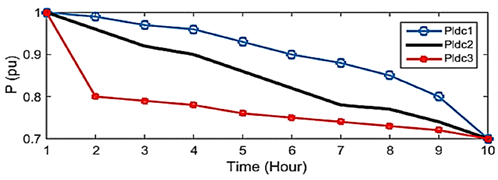Figure 4: Curves with equal index (Hup/Kup) and different (Stdup/Sup)

In peak shaving due to the fact that the peak consumption time is usually limited to several hours (usually up to 2 hours). Hence, another indicator has been defined to show the power difference in peak hours. For this purpose, the average of the first 2 hours of the consumption continuity curve is reduced from the second 2 hours of the curve and is introduced as a useful indicator for peak shaving. This indicator indicates the existence of a significant peak compared to other hours of consumption, which is shown in Equation (5). The greater the difference between the average of these two hours, the more suitable the curve for peak shaving.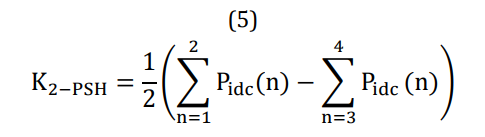Finally, in addition to the two defined indicators, the usage coefficient of each substation UF = Pmax/Pn is also effective in determining the priority. In simpler terms, the higher the relative load of a substation and the closer the nominal consumption is to the nominal value; The sharpening and leveling of the load curve take precedence in that substation because one of the important effects of installing a reserve in a substation is to delay development in that substation, which is very important and economically significant. Finally, the peak index is defined by Equation (6).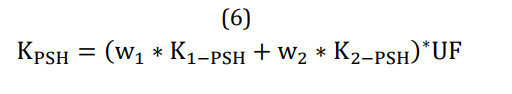4-2- Indicators related to load curve leveling

Unlike peak shaving, which is usually applied over a short period, the load curve leveling can be performed for a longer period. In general, the power can be stored in the battery during the hours when the consumption is less than the average, and the stored power can be delivered to the network during the hours when the consumption is above the average. This is similar to transferring power from valley peaks into valleys. This has many benefits for the network, the most important of which are delaying the development of the substation, making a profit from buying and selling energy, less loading from the transformer, and reducing losses. To level the load curve, you can define an indicator to prioritize the substations, just like a peak shaving. For this purpose, several variables are defined as follows [40-44]:

- m1: The period of the first 8 hours is the continuous consumption curve of the productive period and the average of this 8-hour interval is defined as m1.

- m2: The period of the middle 8 hours of the consumption curve is called the shortcut period and its average is defined as m2.

- m3: The period of the last 8 hours of the consumption curve is called the period of consumption and we consider its average as m3.

In this case, the overnight consumption continuity curve can be summarized by three numbers m1 to m3. The first index of curve leveling is defined by Equation (7):The second index, which somehow indicates a modified standard deviation, is expressed by Equation (8):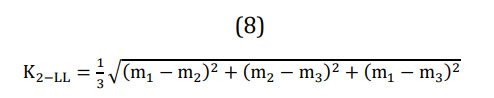Finally, the general index for leveling the load curve is written as Equation (9).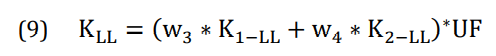The next parameter that can be considered in the problem of load curve leveling is the maximum storage capacity for leveling the load curve completely. If we assume that the storage efficiency is considered 100% and the installation of the storage does not increase the average consumption; In this case, if the storage device can store energy as much as Sup, the curve can be completely flattened. Therefore, the maximum storage energy for leveling the curve is equal to the area of Sup ( ). Therefore, an index called the load level leveling percentage index can be defined as follows:Equation (10) actually indicates that the battery stores a few percent of the rechargeable area. Obviously, if LLPI = 100%, the curve will be perfectly flat. The LLPI index can be used for both peak shaving and load curve leveling. Small values of LLPI (usually less than 0.3) are related to peak shaving and large values are related to curve leveling.

4-3- Cost index

In general, a multi-part cost objective function can be defined in terms of cost, the most important of which are storage costs, maintenance costs, and installation costs. In the discussion of the optimal location of the storage location, due to the same costs related to installations and repairs and maintenance, the cost of the location allocated to the storage is more important. Hence, a cost index in the objective function is considered as follows: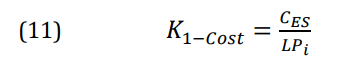Also in the cost index, the average electricity sales index per substation can be defined as follows.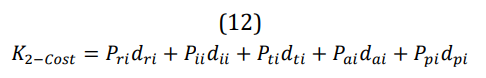This index is provided to include different electricity sales tariffs in the post office. For example, the tariff for the sale of industrial electricity is higher than the tariff for the sale of agricultural electricity. Tariffs related to the sale of energy in terms of per unit in 1993 are shown in Table (1) [44-47].

In relation (12), Pri, Pii, Pti, Pai, and Ppi are the average household, industrial, commercial, agricultural, and general electricity tariffs and the coefficients of dri, dii, dti, dai and dpi, respectively. The corresponding form is related to the percentage of domestic, industrial, commercial, agricultural, and general loads in each substation in the peak consumption hour (study time).

Table (1): Per unit prices of different types of energy sales tariffs

 Homemade General Agriculture Industrial Commercial Type of tariff 1 0.8 0.35 1.05 3 Price

Finally, the cost index can be defined as follows: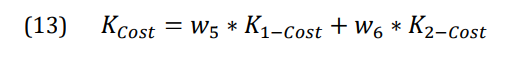Given that the storage installation may take one or more years, it is best to make a medium-term load forecast for the above distribution substations so that the calculations are more accurate. Many methods have been proposed in the articles to predict the load. In this paper, the neural network method along with the sample decision tree has been used for prediction [48-52]. According to Figure (5), the input information is divided into several main categories such as average temperature, average consumption per day, and last week, shutdown or not index, and different days of the week. For training the neural network, the consumption information of the distribution substations in Semnan city in 2014 and 2015 has been used.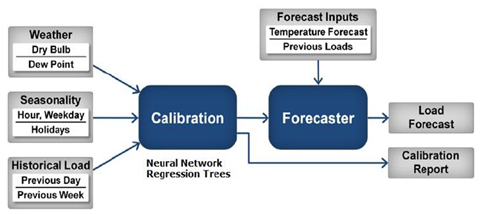Figure 5: Short-term load prediction structure

6- Simulation

The system under study in this article is the distribution network of Semnan city. This network has 10 substations of 63 to 20 kV, the capacities of each of which are between 15 and 40 MVA. Information on the capacity of the substations, the maximum consumption of each substation, the usage rate, and the average annual load growth are given in Table (2). Table (3) shows the information about the percentage of each type of load in each substation and the price of grounding as per unit in each substation.

Table 2: Information on the capacity of transformers in each substation

 Load growth percentage Power factor Profit coefficient Maximum power (MW) Nominal power (MVA) Substation name Substation number 2 0.712 0.391 23.46 2.30 Shargh1 1 # 2 0.783 0.203 24.47 3.40 Shargh2 2 # 1.5 0.505 0.294 8.82 2.15 Foulad fajr 3 # 1.5 0.779 0.325 19.51 2.30 Kolran 4 # 2 0.841 0.465 37.2 2.40 Mirhaj 5 # 2 0.858 0.517 31.04 2.30 Jonoob 6 # 2 0.777 0.421 25.3 2.30 Sorkheh 7 # 3 0.653 0.283 16.98 2.30 Mahdishahr 8 # 1.5 0.564 0.128 3.86 2.15 Chashm 9 # 3 0.685 0.4 6 2.15 Shahmirzad 10#

Table 3: Information on the percentage of loads per substation and land price

 Land prices (PU) Percentage of industrial load Percentage of household load Percentage of agricultural load Percentage of commercial load Percentage of general load Substation number 1.2 40 10 35 0 15 1# 1 50 0 40 0 10 2# 1.4 100 0 0 0 0 3# 1.1 100 0 0 0 0 4# 2.1 0 50 10 30 10 5# 1.8 20 20 40 10 10 6# 0.8 10 25 50 10 5 7# 1.2 10 30 40 15 5 8# 1.2 100 0 0 0 0 9# 1 10 30 40 15 5 10#

As mentioned before, statistical information on consumption in 1393 and 1394 has been used to train the neural network. For the accuracy of the proposed method, information about these two years up to the last week of summer (168 hours) was tested as output. The load prediction results are shown in Figures (6) and (7). As shown in Figure (6), the predicted load is accurately equivalent to the actual consumption. The predicted fault value is shown in Figure (7) and the absolute mean value of fault percentage is equal to 1.41%. According to the results of other papers, this fault value indicates the very good accuracy of the implemented method.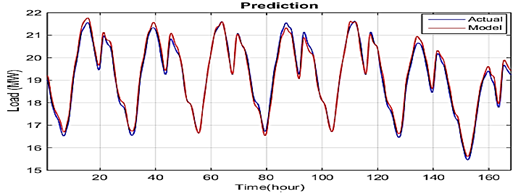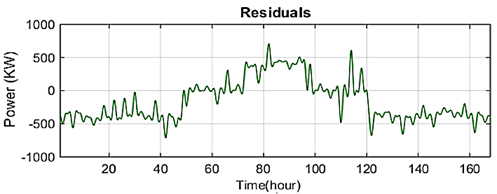Figure 7: Load prediction fault value

To plan the charge and discharge of the storage device, load forecasting has been done for every ten substations under study for the summer of 2016, and the maximum daily consumption of each post has been extracted. As an example, in Figure (8), the predicted time of substation number 6 (south of Semnan) is shown and the amount of peak consumption per day is extracted. As shown in Figure (8), the consumption load in these three months can be easily divided into three groups (working days, part-time days and holidays), which are usually peak consumption on working days and days that Temperature is at its highest, it happens. By forecasting the load of every ten posts under study and extracting information about the peak day, the planning information is completed. Figure (9) shows the consumption curves (per unit) of the substations under study on peak consumption days. The indices described above are calculated based on the information in Figure (9).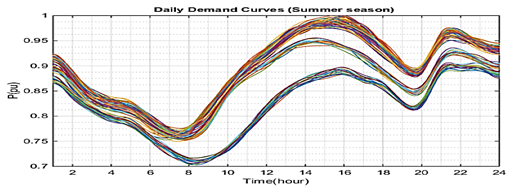Figure 8: Summer weather forecast curves for the South Substation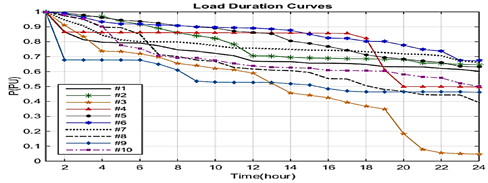Figure 9: Continuity consumption curves of the substations under study

The indicators defined in the paper are calculated individually and finally as a total index for every ten subsatations under study. If we pay attention to the fact that when leveling the load curve completely, the peak shaving also happens automatically, so we can calculate the weight of the load curve leveling indices and cost in calculating the final index. It was considered more than the weight of the peak shaving index. In this paper, to calculate the final index, these coefficients are 40%, 40% and 20%, respectively. According to the calculated indicators, the most suitable post for just peak shaving substation number 7 (Sorkheh substation), the most suitable post for just leveling the load curve of post number 8 (Mahdishahr substation) and the most suitable post from In terms of only reducing the cost of post number 5 (Shahid Mirhaj substation). According to the calculated final index, the most suitable substation for installing a storage device considering all three indicators is substation number 5 (Shahid Mirhaj substation). Two conditions must be considered for optimal storage storage planning. First, the installation of a storage device should not increase the average consumption in the substation, and secondly, it should be a priority to discharge during peak consumption hours. The first step in planning is to determine the maximum storage capacity and determine its maximum output power. As mentioned, the maximum storage capacity is equal to the Sup index, in which case the LLPI index will be 100%. For smaller LLPI values, the storage capacity will be calculated according to Equation (10). Due to the fact that the average consumption of the substation does not increase, the amount of maximum storage power is equal to the maximum difference between the consumption curve and the average amount. The results of optimal charge and discharge planning of substation No. 5 (Shahid Mirhaj) are shown in Figures (10) to (19) for different LLPI values.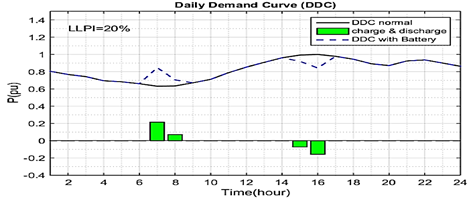Figure 10: Daily consumption curve before and after battery installation for LLPI = 0.2